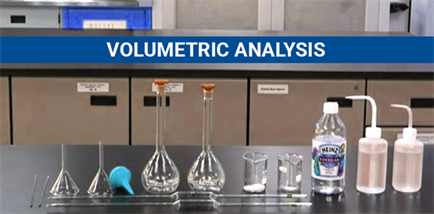# Volumetric Analysis## What is Volumetric Analysis?

Volumetric analysis is a quantitative analytical method which is used widely. As the name suggests, this method involves measurement of the volume of a solution whose concentration is known and applied to determine the concentration of the analyte.

In other words, measuring the volume of a second substance that combines with the first in known proportions is known as Volumetric analysis or titration. It is this method of quantitative analysis that allows us to determine the concentration of the analyte. The first method of Volumetric Analysis was devised and found by the French chemist Jean-Baptiste-Andre-Dumas; as he was trying to determine the proportion of nitrogen combined with other elements in organic compounds. To ensure the conversion of the nitrogen compound into pure gas, the nitrogen compound was burnt in a furnace and passed along a furnace in a stream of carbon dioxide that is passed into a strong alkali solution. The mass of the nitrogen is calculated and occupies under known conditions of pressure and volume from the sample.

### Procedure for Volumetric Analysis

1. A typical titration starts with a beaker or flask containing a precise volume of the analyte and small amount of indicator placed underneath a calibrated burette or pipette containing the titrant.
2. The solution that needs to be analysed needs to have an accurate weighed in the sample of +/- 0.0001g of the material to be analysed.
3. Choosing the right kind of material to be analysed is also very important, as choosing the wrong type of titrant will give us the wrong results. A substance that reacts rapidly and completely to produce a complete solution is chosen.
4. Small quantities of titrant are added to the analyte and indicator till the indicator changes colour in reaction to the titrant saturation threshold reflects the arrival at the endpoint of the titration.
5. The titration has to be continued up until the reaction is complete and the amount of reactant added is exactly the amount that is needed to complete the reaction.
6. Another important step is in measuring the right volume of the standard solution since molarity is a standard metric to calculate the number of moles present in a solution.
7. Based on the desired endpoint, single drops or less than a drop of the titrant makes a difference between a permanent and temporary change in the indicator.
8. If the reagent or reactant that we use is to be made into a standard solution then we can weigh and dissolve the reagent into a solution, so that it is in a definitive volume within a volumetric flask.

### Basic principles of volumetric analysis

1. The solution to be analysed contains an unknown amount of chemicals.
2. The reagent of unknown concentration reacts with a chemical of an unknown amount in the presence of an indicator (mostly phenolphthalein) to show the end-point. It’s the point indicating the completion of the reaction.
3. The volumes are measured by titration which completes the reaction between the solution and reagent.
4. The volume and concentration of reagent which are used in the titration show the amount of reagent and solution.
5. The amount of unknown chemical in the specific volume of solution is determined by the mole fraction of the equation.

When the endpoint of the reaction is reached, the volume of reactant consumed is measured and applied to carry volumetric analysis calculations of the analyte by the following formula,

Ca= Ct Vt M / Va

Where,

Ca is the analyte concentration, typically in molarity.

Ct is the titrant concentration, typically in molarity.

V is the volume of the titrant which is used, typically in liters.

M is the mole ratio of the analyte and reactant from the balanced equation.

V is the volume of the analyte, typically in liters.

Many non-acid-base titrations are needed a constant pH throughout the reaction. Therefore, a buffer solution can be added to the titration chamber to maintain the pH value.

Test Your Knowledge On Volumetric Analysis!

#### 1 Comment

1. Noah kipkemoi